• +91 9971497814
• info@interviewmaterial.com

# RD Chapter 22- Brief Review of Cartesian System of Rectangular Coordinates Ex-22.2 Interview Questions Answers

### Related Subjects

Question 1 : Find the locus of a point equidistant from the point (2, 4) and the y-axis.

Let P (h, k) be any point on the locus and let A (2, 4) and B(0, k).

Then, PA = PB

PA2 = PB2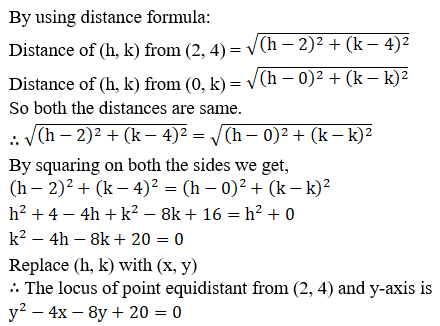Question 2 : Find the equation of the locus of a point which moves such that the ratio of its distance from (2, 0) and (1, 3) is 5: 4.

Let P (h, k) be any point on the locus and let A (2, 0) and B(1, 3).

So then, PA/ BP = 5/4

PA2 = BP2 = 25/16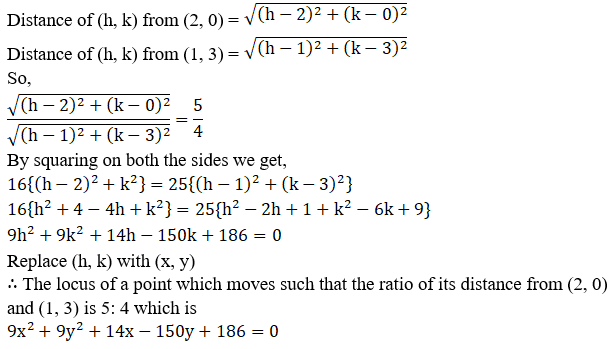Question 3 :
A point moves as so that the difference of its distances from (ae, 0) and (-ae, 0) is 2a, prove that the equation to its locus is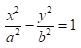, where b2 = a2 (e2 – 1).

Let P (h, k) be any point on the locus and let A (ae, 0) and B(-ae, 0).

Where, PA – PB = 2a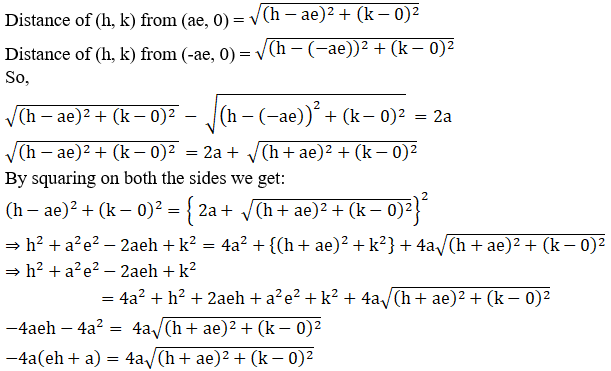Now again let us square on both the sides we get,

(eh + a)2 = (h +ae)2 + (k – 0)2

e2h2 + a2 +2aeh = h2 + a2e2 +2aeh + k2

h2 (e2 – 1) – k2 =a2 (e2 –1)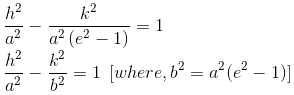Now let us replace (h, k) with (x, y)

The locus of a point such that the difference of its distancesfrom (ae, 0) and (-ae, 0) is 2a.Where b2 = a(e2 –1)

Hence proved.

Question 4 : Find the locus of a point such that the sum of its distances from (0, 2) and (0, -2) is 6.

Let P (h, k) be any point on the locus and let A (0, 2) and B (0, -2).
Where, PA – PB = 6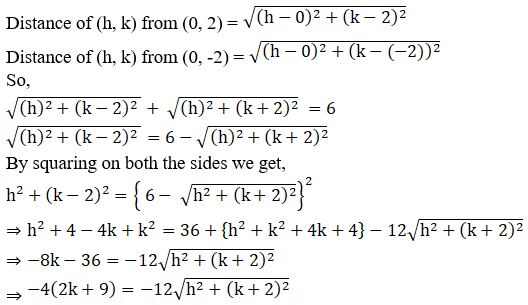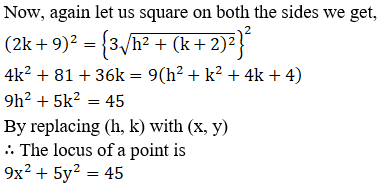Question 5 : Find the locus of a point which is equidistant from (1, 3) and x-axis.

Let P (h, k) be any point on the locus and let A (1, 3) and B (h, 0).
Where, PA = PB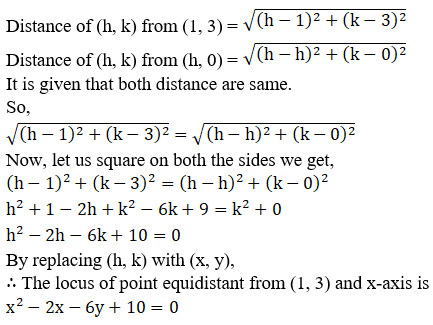Question 6 : Find the locus of a point which moves such that its distance from the origin is three times is distance from x-axis.

Let P (h, k) be any point on the locus and let A (0, 0) and B (h, 0).
Where, PA = 3PB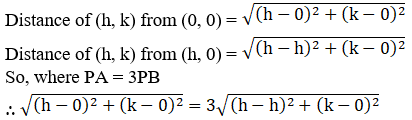Now by squaring on both the sides we get,

h2 + k2 = 9k2

h2 = 8k2

By replacing (h, k) with (x, y)

Thelocus of point is x2 = 8y2

Todays Deals### RD Chapter 22- Brief Review of Cartesian System of Rectangular Coordinates Ex-22.2 Contributorskrishan

Name:
Email:

# Latest News# 9000 interview questions in different categories